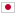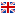Contest Duration: - (local time) (300 minutes)
J - Farm Village/Time Limit: 2 sec / Memory Limit: 512 MB

### Problem Statement

There is a village along a road. This village has $N$ houses numbered $1$ to $N$ in order along the road. Each house has a field that can make up to two units of the crop and needs just one unit of the crop. The total cost to distribute one unit of the crop to each house is the summation of carrying costs and growing costs.

• The carrying cost: The cost to carry one unit of the crop between the $i$-th house and the $(i+1)$-th house is $d_i$. It takes the same cost in either direction to carry.
• The growing cost: The cost to grow one unit of the crop in the $i$-th house's field is $g_i$.

Your task is to calculate the minimum total cost to supply one unit of the crop to each house.

### Input

The input consists of a single test case formatted as follows.

$N$ $d_1 \ldots d_{N-1}$ $g_1 \ldots g_N$

The first line consists of an integer $N \ (2 \le N \le 200{,}000)$, which is the number of the houses. The second line consists of $N-1$ integers separated by spaces. The $i$-th integer $d_i \ (1 \le d_i \le 10^9, 1 \le i \le N-1)$ represents the carrying cost between the $i$-th and the $(i+1)$-th houses. The third line consists of $N$ integers separated by spaces. The $i$-th integer $g_i \ (1 \le g_i \le 10^9, 1 \le i \le N)$ represents the growing cost of the $i$-th house's field.

### Output

Print the minimum cost to supply one unit of the crop to each house.

### Sample Input 1

2
3
1 5


### Output for Sample Input 1

5


### Sample Input 2

3
100 100
1 2 3


### Output for Sample Input 2

6


### Sample Input 3

4
1 2 3
1 1 100 100


### Output for Sample Input 3

12